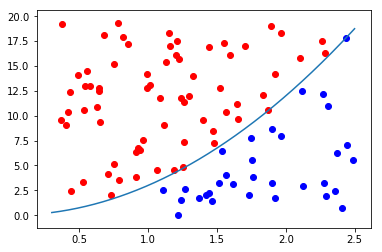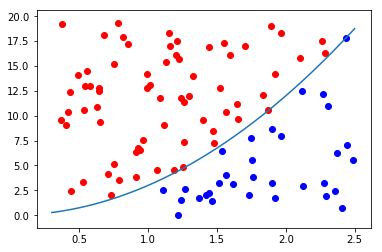### Monte Carlo Integration in PythonMy last post was pretty intense, so I thought that I would drop back down to something a little bit simpler for this post, before I go off and start in on the March Madness stuff again. It turns out that writing that last post really kicked my butt, so I’m taking a bit of a break. I want to talk about what we did right at the end of that post. What we did is we ran some simulations.

It turns out that doing simulations is a really useful thing. Like we saw last week, we can use them to figure out what the outcome of an event will be. The concrete example that we had in the last post was the simulation of a basketball game. The number one thing that you need when you are doing a simulation like this is a probability distribution from which to draw. The way we did that was by estimating a bayesian model.

Today, because I want to do something simple, I want to write a post about doing some calculus for integration. It turns out that in some instances we can’t compute an integral. It is just downright hard. But we can cheat for numerical integration! In fact this cheat is the only way we can estimate multinomial probit models, that get around the whole independence of irrelevant alternatives assumption in logistic regression. (Note, there are other ways to get around it like mixed logit models).

The way that we cheat is by randomly sampling the space around the function. This gives us a technique called Monte Carlo integration. And it is very much related to the idea of Monte Carlo Markov Chains, which let us do the Bayesian stuff in the first place. What we have with this method is a very simple approach to integration. So I thought that I would demonstrate the technique.

### On The Functions On Which to use Monte Carlo Integration

So it really doesn’t make sense to use this technique on easy functions that have a numerical solution which is easy to calculate. It does help in verifying that the answers that it gives you are reasonable. It is also worth noting that this procedure only produces approximate answers. You have to give the monte carlo aspect a really long time to run, or else you get very poor results.

Okay, so we’ll be running this Monte Carlo integration on two functions which I will define with python in the code below:

import numpy as np
import matplotlib.pyplot as plt

def easy_function(x):
return((3)*(x**2))

def hard_function(x):
return((1/np.sqrt(2*np.pi))*np.exp(-(x**2)/2))


Okay, so our “easy function” is a polynomial and is relatively easy to compute the definite integral by hand. The hard function is the standard normal function, with mean 0 and standard deviation of 1, there is no closed form solution to this function. We can take a look at what these functions look like with this code for matplotlib:

X=np.linspace(-20,20,1000)
plt.plot(X,easy_function(X))
plt.show()

plt.plot(X,hard_function(X))
plt.show()


Which gives you the following two figures:### Now How do you do Monte Carlo Integration

Monte Carlo integration is very easy to do. Here is the nuts and bolts of the procedure. Look at an area of interest, and make sure that the area contains parts that are above the highest point of the graph and the lowest point on the graph of the function that you wish to integrate. Cool, now you have a rectangle whose area is known that contains the area under the function of interest, in other words the area under the function is some fraction of the area of the rectangle for the region that you are looking at.

So now all we need to do is to find some way to calculate the proportion of the area of the rectangle that is also underneath the function. The way that we do that is by randomly throwing darts at the area. And simply counting the number of darts that land underneath the function and how many are above the function. The proportion that land underneath the function will be roughly equivalent to the proportion of the area under the curve.

We then multiply this proportion by the area of the rectangle and there is our approximate answer. The more darts that you throw, the better your answer will be. This is such a dumb technique for computing definite integrals an ambitious college student should be able to design a computer program that can do it.

Here is the code to do what I just described:

def integrate(x1,x2,func=easy_function,n=100000):
X=np.linspace(x1,x2,1000)
y1=0
y2=max((func(X)))+1
print(x1,x2,y1,y2)
area=(x2-x1)*(y2-y1)
check=[]
xs=[]
ys=[]
for i in range(n):
x=np.random.uniform(x1,x2,1)
xs.append(x)
y=np.random.uniform(y1,y2,1)
ys.append(y)
if abs(y)>abs(func(x)) or y<0:
check.append(0)
else:
check.append(1)
print(np.mean(check))
return(np.mean(check)*area,xs,ys)

print(integrate(0.3,2.5))
print(integrate(0.3,2.5,hard_function))


This last little bit of code will let you visualize what is going on. Basically, we take the proportion of blue dots to the total number of dots and multiply by the area of the viewing window for this graph. To get the estimate of the area under the curve. For the parabola, “easy function”, I got an answer of 15.628, which is really close to the true value of 15.598. Certainly, if I included more samples I would be closer. As for the “hard function”, this procedure gave me an estimate of the area under the curve of 0.380, which seems reasonable to me, for a standard normal distribution (I’m not going to check it). Here is the code to visualize the process:

_,x,y,c=integrate(0.3,2.5,n=100)
df=pd.DataFrame()
df['x']=x
df['y']=y
df['c']=c

X=np.linspace(0.3,2.5,1000)
plt.plot(X,easy_function(X))
plt.scatter(df[df['c']==0]['x'],df[df['c']==0]['y'],color='red')
plt.scatter(df[df['c']==1]['x'],df[df['c']==1]['y'],color='blue')
plt.show()


Which will produce this graph:### Just give me the Code!

Here is the full code:

#!/usr/bin/env python3
# -*- coding: utf-8 -*-
"""
Created on Sat Jul 15 10:43:57 2017

@author: ryan
"""

import numpy as np
import matplotlib.pyplot as plt
import pandas as pd

def easy_function(x):
return((3)*(x**2))

def hard_function(x):
return((1/np.sqrt(2*np.pi))*np.exp(-(x**2)/2))

X=np.linspace(-20,20,1000)
plt.plot(X,easy_function(X))
plt.show()

plt.plot(X,hard_function(X))
plt.show()

def integrate(x1,x2,func=easy_function,n=100000):
X=np.linspace(x1,x2,1000)
y1=0
y2=max((func(X)))+1
print(x1,x2,y1,y2)
area=(x2-x1)*(y2-y1)
check=[]
xs=[]
ys=[]
for i in range(n):
x=np.random.uniform(x1,x2,1)
xs.append(x)
y=np.random.uniform(y1,y2,1)
ys.append(y)
if abs(y)>abs(func(x)) or y<0:
check.append(0)
else:
check.append(1)
return(np.mean(check)*area,xs,ys,check)

print(integrate(0.3,2.5))
print(integrate(0.3,2.5,hard_function))
_,x,y,c=integrate(0.3,2.5,n=100)
df=pd.DataFrame()
df['x']=x
df['y']=y
df['c']=c

X=np.linspace(0.3,2.5,1000)
plt.plot(X,easy_function(X))
plt.scatter(df[df['c']==0]['x'],df[df['c']==0]['y'],color='red')
plt.scatter(df[df['c']==1]['x'],df[df['c']==1]['y'],color='blue')
plt.show()